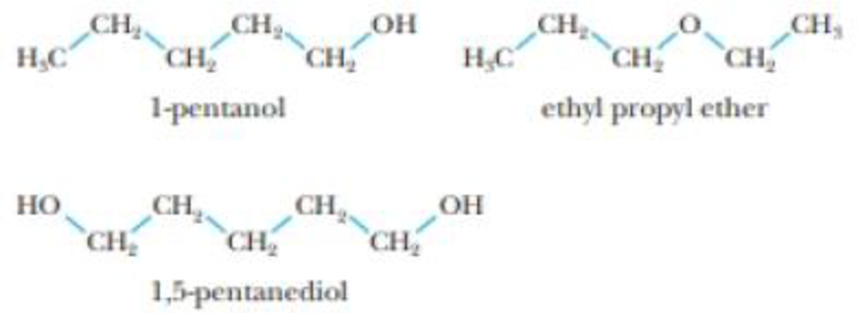Chapter 11.6, Problem 1.1ACP

Chapter
Section
Textbook Problem

Assume that a mixture of the three molecules below are separated on a C-18 column using a methanol/water mixture as the mobile phase. a. Which of the three molecules is most attracted to the mobile phase? What are the forces that attract the molecule to the water/methanol phase? b. Which molecule is most attracted to the stationary phase? What are the forces that attract the molecule to the nonpolar phase? c. In what order will the three molecules exit (or “elute from”) the column?(a)

Interpretation Introduction

Interpretation:

Mixture of three molecules are separated on a C18 column using methanol/water as the mobile phase, following answer is should be identified.

Concept Introduction:

London dispersion force also called an induced dipole-induced dipole attraction is a temporary attractive force that results when the electrons in two adjacent atoms occupy positions that make the atoms form temporary dipoles.

Dipole-dipole interaction results when two dipolar molecules interact with each other through space, there occurs a partially negative charge of one of the polar molecules is attracted to the partially positive charge of the second polar molecule.

Ion-dipole interaction results of an electrostatic interaction between a charged ion and a molecule that has a dipole.

A hydrogen bonding is partially an electrostatic attraction between H atom which is bonded to more electronegative atom such as N, O, orF and another adjacent atom bearing a lone pair of electron.

Explanation

In one common form of chromatography called partition chromatography both mobile and stationary phase are liquids. The simple example a polar mobile phase is passed through a very thin column containing a non-polar stationary phase.

(b).

Interpretation Introduction

Interpretation:

It should be determine that which molecules is most attracted to the stationary phase, and what are the forces that attract the molecule to the non-polar phase.

Concept Introduction:

London dispersion force also called an induced dipole-induced dipole attraction is a temporary attractive force that results when the electrons in two adjacent atoms occupy positions that make the atoms form temporary dipoles.

Dipole-dipole interaction results when two dipolar molecules interact with each other through space, there occurs a partially negative charge of one of the polar molecules is attracted to the partially positive charge of the second polar molecule.

Ion-dipole interaction results of an electrostatic interaction between a charged ion and a molecule that has a dipole.

A hydrogen bonding is partially an electrostatic attraction between H atom which is bonded to more electronegative atom such as N, O, orF and another adjacent atom bearing a lone pair of electron.

(c).

Interpretation Introduction

Interpretation:

It should be determine that which molecules is most attracted to the stationary phase, and what are the forces that attract the molecule to the non-polar phase.

Concept Introduction:

London dispersion: This force also called an induced dipole-induced dipole attraction is a temporary attractive force that results when the electrons in two adjacent atoms occupy positions that make the atoms form temporary dipoles.

Dipole-dipole interaction: Dipole interaction results when two dipolar molecules interact with each other through space, there occurs a partially negative charge of one of the polar molecules is attracted to the partially positive charge of the second polar molecule.

Ion-dipole interaction results of an electrostatic interaction between a charged ion and a molecule that has a dipole.

A hydrogen bonding is partially an electrostatic attraction between H atom which is bonded to more electronegative atom such as N, O, orF and another adjacent atom bearing a lone pair of electron.

Still sussing out bartleby?

Check out a sample textbook solution.

See a sample solution

The Solution to Your Study Problems

Bartleby provides explanations to thousands of textbook problems written by our experts, many with advanced degrees!

Get Started

Does the cell cycle refer to mitosis as well as meiosis?

Human Heredity: Principles and Issues (MindTap Course List)

Why did the Copernican hypothesis win gradual acceptance?

Horizons: Exploring the Universe (MindTap Course List)

What does the acronym MUFA stand for?

Organic And Biological Chemistry